# Quarks

A LevelAS LevelAQA

## Quarks

A fundamental particle that make up hadrons, quarks come in six flavours, thr of which you need to know about: up, down and strange.

## Properties of Quarks

Quarks and antiquarks are fundamental particles and are the building blocks of hadrons. Antiquarks make up the antiparticles of hadrons.

There are three types of quark you need to know: up, down and strange.

All quarks have a baryon number of $\dfrac{1}{3}$ and all antiquarks have a baryon number of $-\dfrac{1}{3}$. They also all have a value for charge with the up quark having a charge of $+\dfrac{2}{3}$ and both down and strange having a charge of $-\dfrac{1}{3}$.

Strange quarks have an additional property called strangeness. It is a quantum number, like baryon and lepton numbers.

Antiquarks have the opposite charge, baryon number and strangeness to their respective quarks.

A particle’s baryon number, charge and strangeness can be found by adding the values for each of the quarks together.

 Quark Symbol Charge Baryon Number Strangeness up $u$ $+\dfrac{2}{3}$ $+\dfrac{1}{3}$ 0 down $d$ $-\dfrac{1}{3}$ $+\dfrac{1}{3}$ 0 strange $s$ $-\dfrac{1}{3}$ $+\dfrac{1}{3}$ -1 anti-up $\bar{u}$ $-\dfrac{2}{3}$ $-\dfrac{1}{3}$ 0 anti-down $\bar{d}$ $+\dfrac{1}{3}$ $-\dfrac{1}{3}$ 0 anti-strange $\bar{s}$ $+\dfrac{1}{3}$ $-\dfrac{1}{3}$ +1
A LevelAS LevelAQA

## Baryons

Baryons are made up of $3$ quarks and antibaryons are made up of $3$ antiquarks.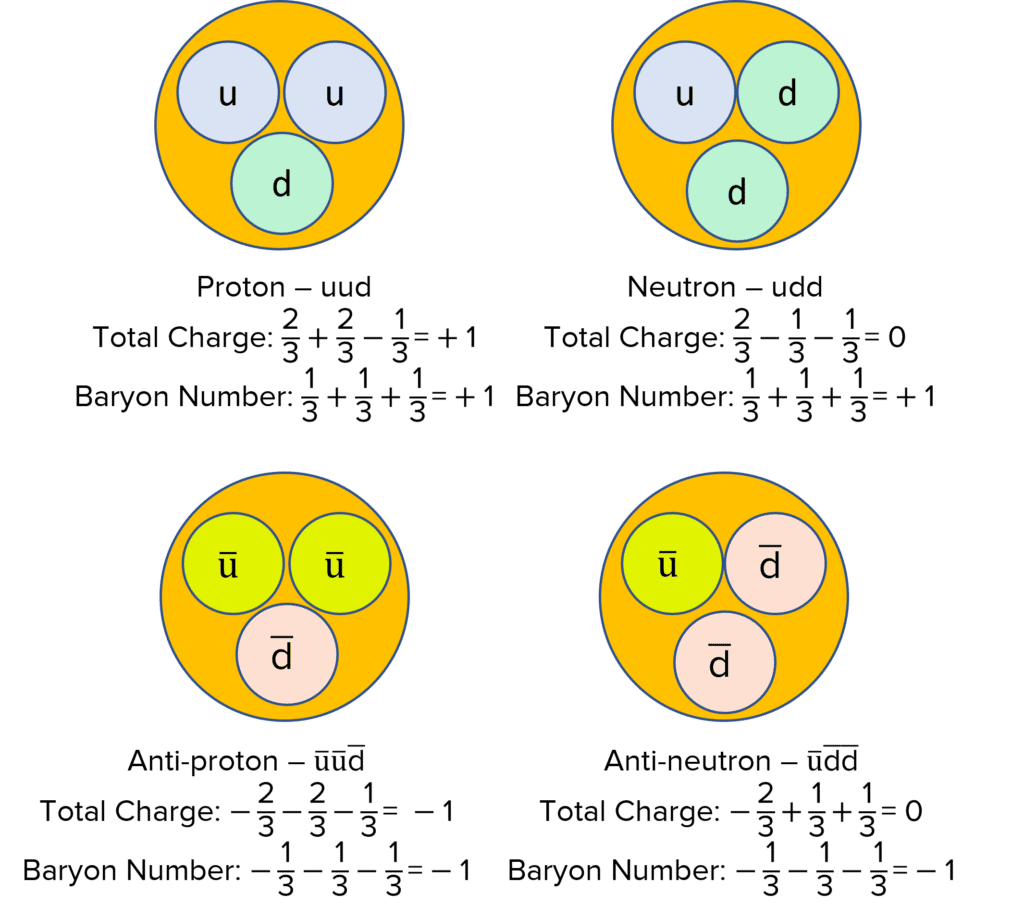Protons are comprised of $2$ up quarks and $1$ down quark, meanwhile neutrons have $1$ up quark and $2$ down quarks. By combining the properties of the quarks, we get the distinctive properties of the proton and neutron.

Quarks were first discovered through firing high energy electrons at protons. By observing how these electrons scattered, scientists determined there must be $3$ concentrations of charge inside the proton.

A LevelAS LevelAQA

## Mesons

Mesons contain $2$ quarks, this is always a combination of a quark and an antiquark.

Pions are a type of meson and are made up from a combination of up, down, anti-up and anti-down quarks.

$\pi^+$ and $\pi^-$ are antiparticles of each other. The antiparticle of $\pi^0$ is simply itself, $\pi^0$.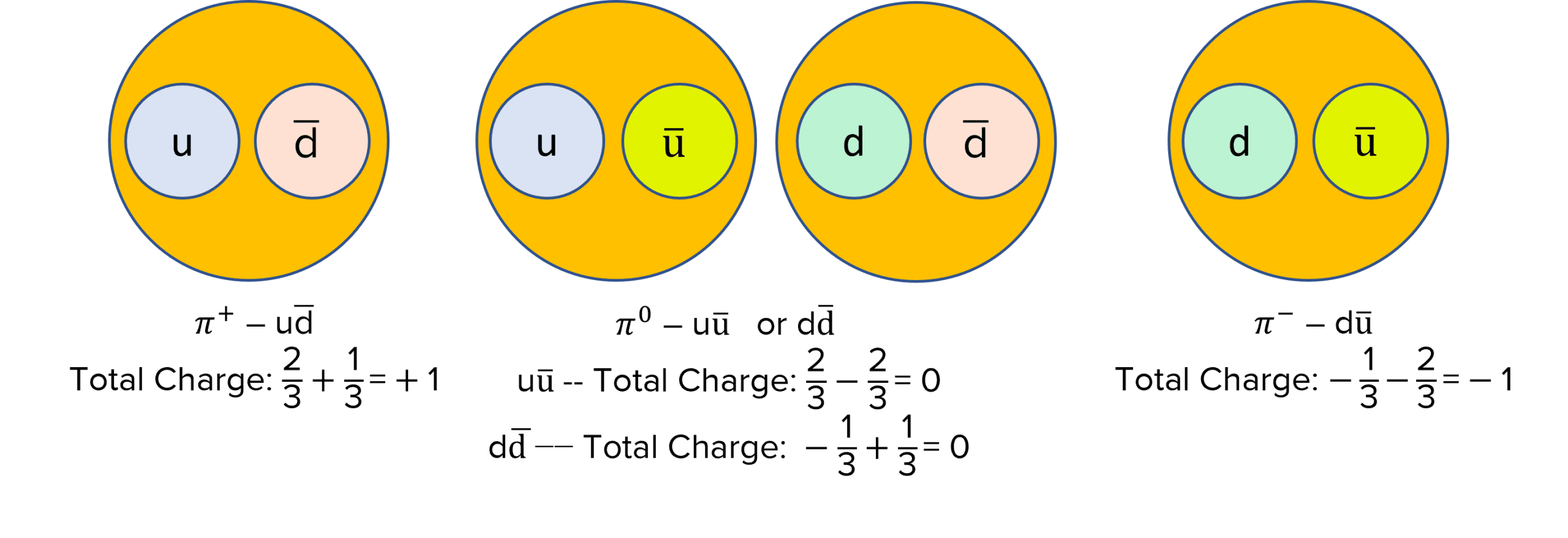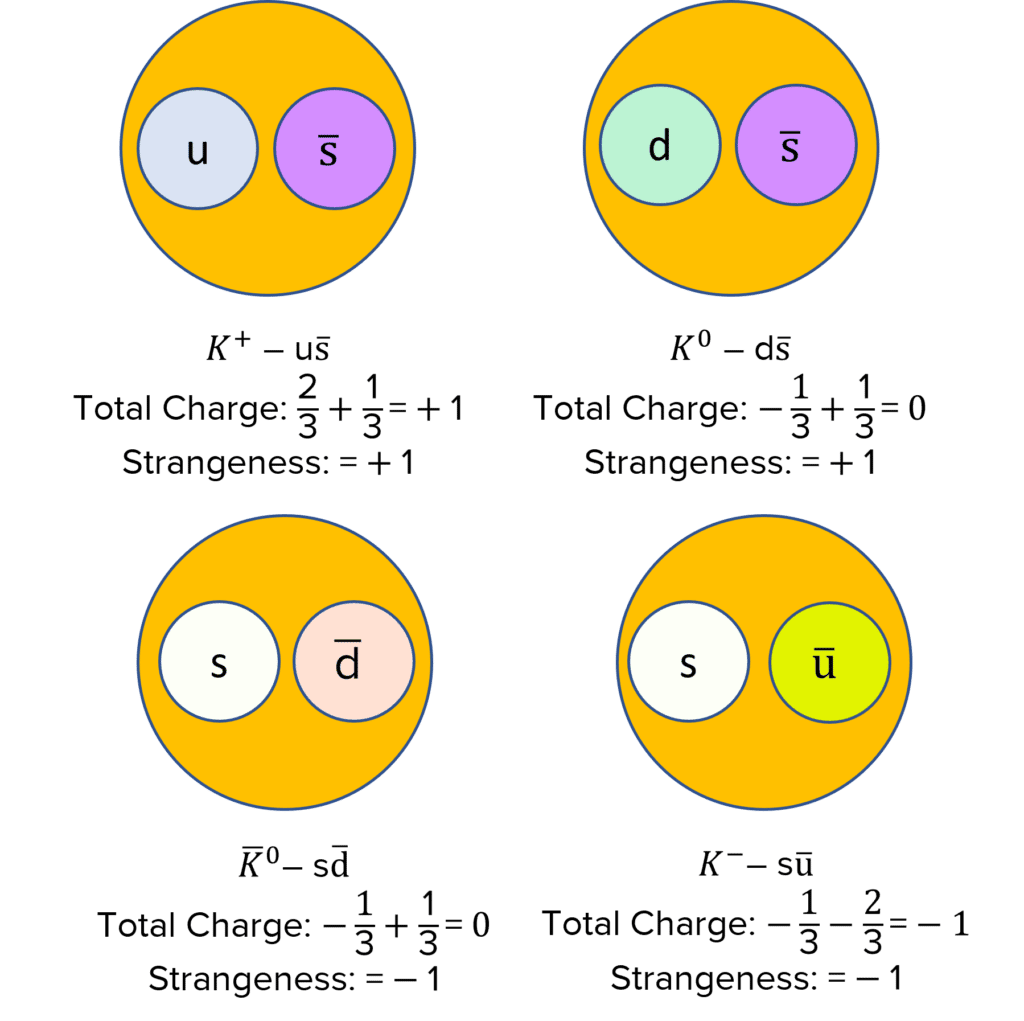All kaons have either a strange or an anti-strange quark. Strange quarks are paired with either an up antiquark or down antiquark and anti-strange quarks are paired with an up or down quark.

Because kaons have a strange quark, they also have a strangeness as a property.

A LevelAS LevelAQA

## Strangeness

Strange quarks are found in kaons. They include the strange and anti-strange quarks. All strange particles have a property of strangeness, which is a quantum number.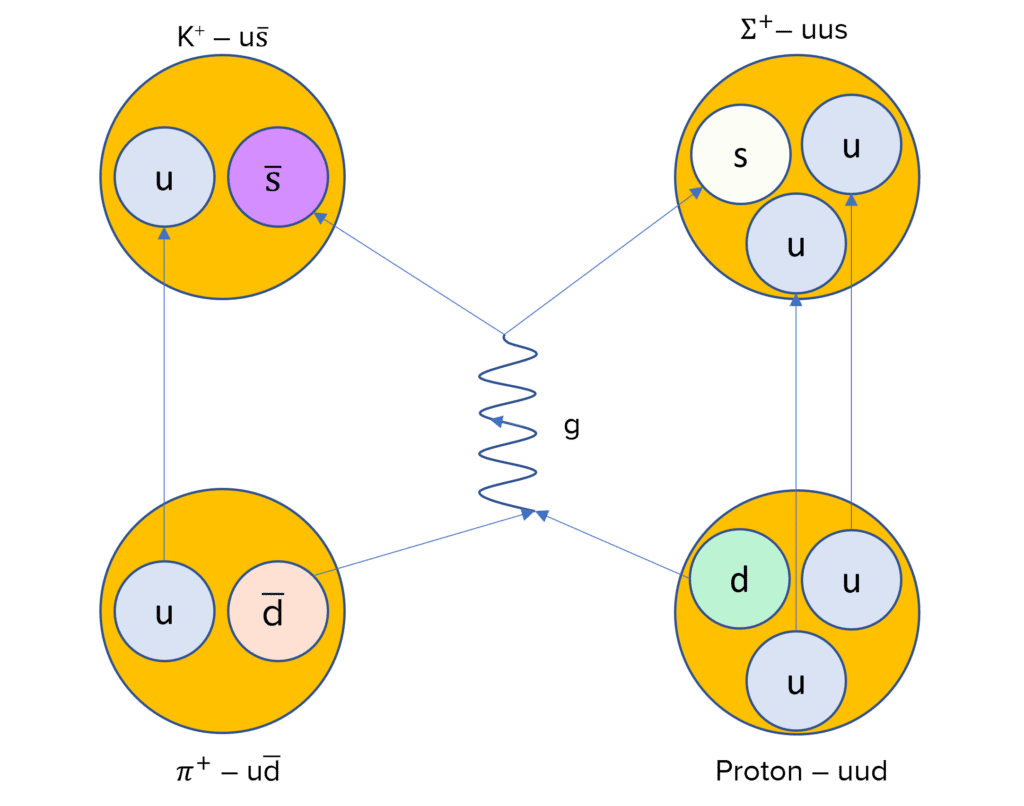Strange particles are produced through the strange interaction. In this interaction, strangeness is conserved. Therefore, to conserve strangeness, strange particles are produced in pairs.

An example of the production of a strange particle is the interaction between a positive pion and a proton. This interaction involves the strong interaction and the gluon $\left(g\right)$ acts as the exchange particle. The equation and  Feynman diagram below shows this interaction:

$\pi^+ + p \: \rightarrow \: K^+ + \Sigma^+$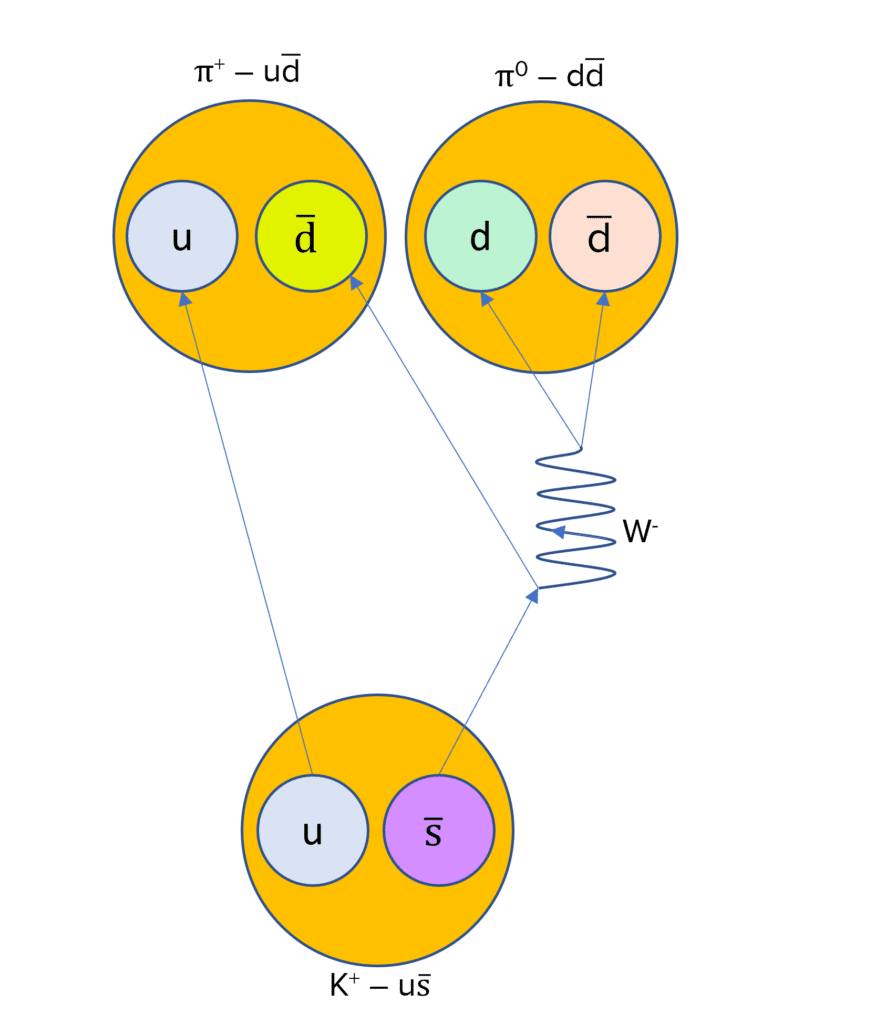Kaons decay through the weak interaction. An example of a kaon decay is the decay of a positive kaon into $2$ neutral pions. This involves the W boson, which is the exchange particle for the weak interaction. The following diagram shows the Feynman diagram of the interaction.

$K^+ \rightarrow \: \pi^+ + \pi^0$

A LevelAS LevelAQA

## Changing Quark Type

Quarks are able to change type through the weak interaction. These can be seen in the beta decays.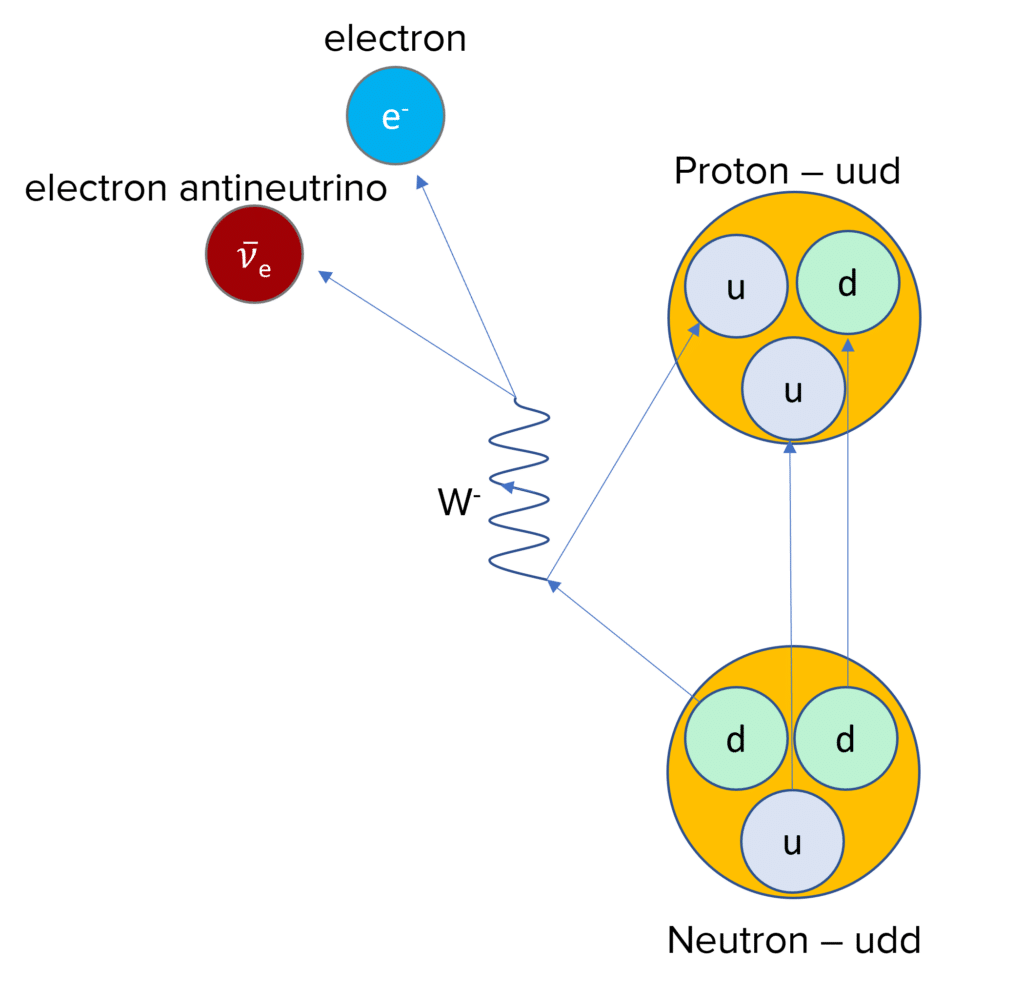In beta-minus decay, a neutron is converted to a proton through the changing of a down quark into an up quark. This will involve the exchange of a $W^{-}$ boson and produces an electron and electron antineutrino:

$n \: \rightarrow \: p + e^- + \bar{v}_e$

In terms of quarks, the interaction can be written as:

$d \: \rightarrow \: u + e^- + \bar{v}_e$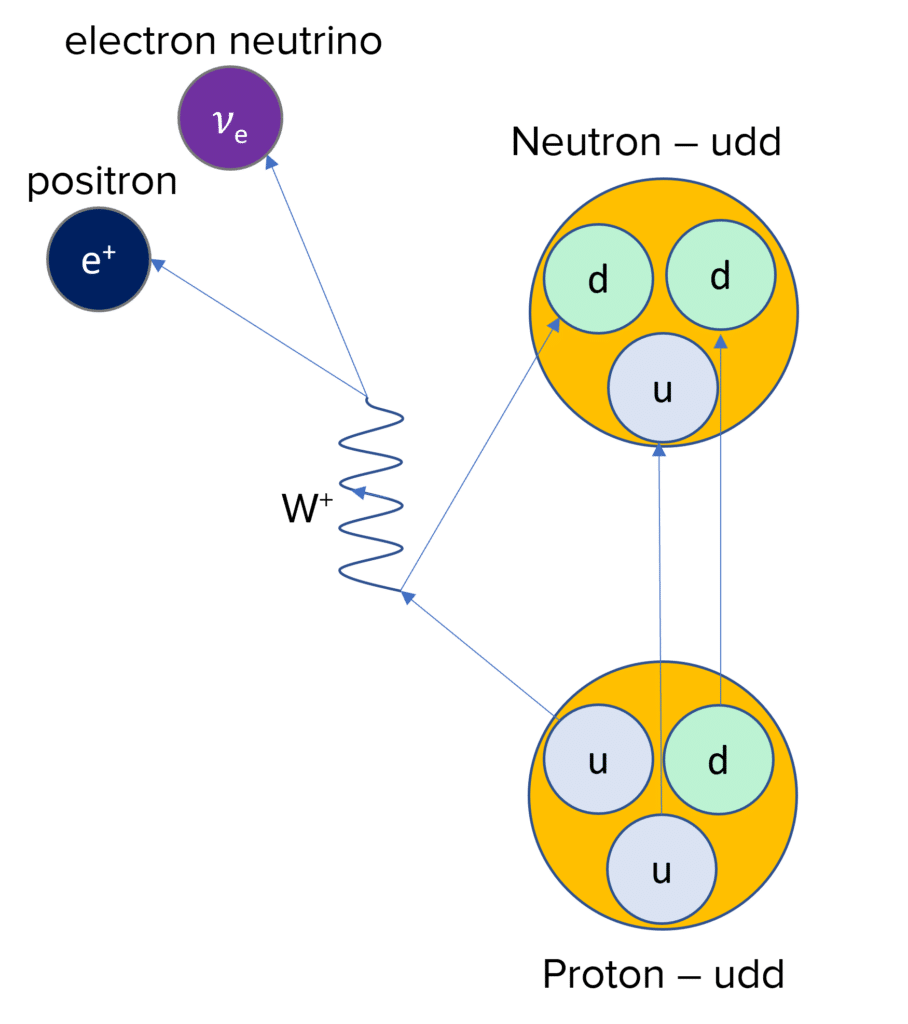In beta-plus decay, a proton changes to a neutron and a positron and electron neutrino are released. This will involve an up quark changing to a down quark, which involves the exchange of a $W^+$ boson.

$p \: \rightarrow \: n + e^+ + v_e$

In terms of quarks, the interaction can be written as:

$u \: \rightarrow d + e^+ + v_e$

A LevelAS LevelAQA

## Free Quarks

It is not possible for quarks to exist in isolation. Even if you gave a particle enough energy to split the quarks apart, the excess energy would produce a quark and antiquark pair through pair production, which would produce a meson. This phenomenon is called quark confinement.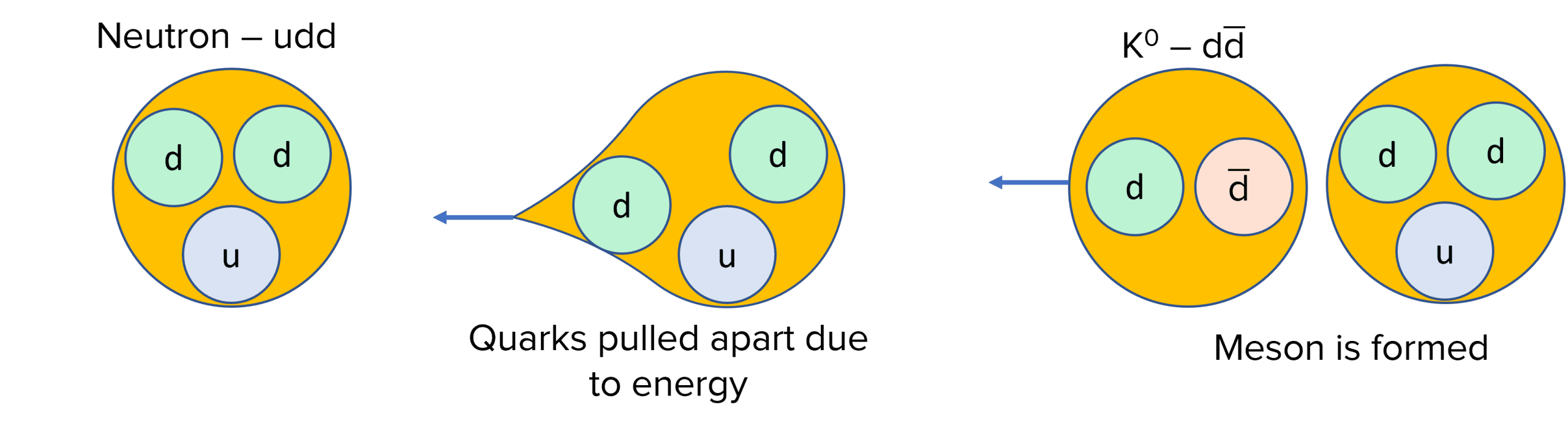A LevelAS LevelAQA

## Quarks Example Questions

A. $\bar{u} \bar{d} \bar{d}$

B. $d \bar{d}$

C. $s \bar{u}$

Two strange particles are produced of opposite strangeness to ensure the overall strangeness is zero.

If energy is applied to a particle to try and split the quark, the energy is converted into the creation of 2 new quarks, a quark and antiquark pair. This process is called quark confinement.

## You May Also Like...### MME Learning Portal

Online exams, practice questions and revision videos for every GCSE level 9-1 topic! No fees, no trial period, just totally free access to the UK’s best GCSE maths revision platform.

£0.00